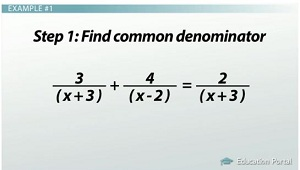# How to Solve a Rational Equation

An error occurred trying to load this video.

Try refreshing the page, or contact customer support.

Coming up next: Rational Equations: Practice Problems

### You're on a roll. Keep up the good work!

Replay
Your next lesson will play in 10 seconds
• 0:05 Rational Equation
• 1:34 Example #1
• 4:44 Example #2
• 7:38 Lesson Summary

Want to watch this again later?

Timeline
Autoplay
Autoplay
Speed

#### Recommended Lessons and Courses for You

Lesson Transcript
Instructor: Kathryn Maloney

Kathryn teaches college math. She holds a master's degree in Learning and Technology.

A rational equation is one that contains fractions. Yes, we will be finding a common denominator that has 'x's. But no worries! Together we will use a process that will help us solve rational equations every time!

## Rational Equations

A rational equation is an equation that contains fractions with xs in the numerator, denominator or both. Here is an example of a rational equation: (4 / (x + 1)) - (3 / (x - 1)) = -2 / (x^2 - 1).

Let's think back for a moment about solving an equation with a fraction. 1/3 x = 8. We think of the 3 in the denominator as being a prisoner, and we want to release it. To set the 3 free, we multiply both sides of the equation by 3. Think of it as 3 letting both sides of the equation know he's leaving. 3 (1/3 x) = 8 (3).

This process freed our denominator and got rid of the fraction - x = 24. It is also the process we use to solve rational equations with one extra step. In rational equations, sometimes our solution may look good, but they carry a virus; that is, they won't work in our equation. These are called extraneous solutions. The steps to solve a rational equation are:

1. Find the common denominator.
2. Multiply everything by the common denominator.
3. Simplify.
4. Check the answer(s) to make sure there isn't an extraneous solution.

Let's solve a couple together.

## Example #1

Example number one: solve. Remember to check for extraneous solutions. (3 / (x + 3)) + (4 / (x - 2)) = 2 / (x + 3).

Our first step is to figure out the terms that need to be released from the denominators. I look at 3 / (x + 3). I write down (x + 3) as one of my common denominators. I look at 4 / (x - 2). I write down (x - 2) as another part of my common denominator. I look at 2 / (x + 3). Since I already have (x + 3) written in my denominator, I don't need to duplicate it.

Next, we multiply everything by our common denominator - (x+3)(x-2). This is how that will look: ((3(x + 3)(x - 2)) / (x + 3)) + ((4(x + 3)(x - 2)) / (x - 2)) = (2(x + 3)(x - 2)) / (x + 3))

It isn't easy for the denominators to be released; there is a battle, and like terms in the numerator and denominator get canceled (or slashed). Slash (or cancel) all of the (x + 3)s and (x - 2)s in the denominator and numerator. Our new equation looks like: 3(x - 2) + 4(x + 3) = 2(x - 2).Distribute to simplify: (3x - 6) + (4x + 12) = 2x - 4. Collect like terms and solve. 3x + 4x = 7x, -6 + 12 = 6. We end up with 7x + 6 = 2x - 4.

Subtract 2x from both sides: 7x - 2x = 5x. Subtracting from the other side just cancels out the 2x, and we get 5x + 6 = -4. Subtract 6 from both sides: -4 - 6 = -10. Again, subtracting 6 will cancel out the +6, so we end up with 5x = - 10. Divide by 5 on both sides, and we cancel out the 5 and give us x = - 2. It turns out x = - 2.

The reason we check our answers is that sometimes we get a virus, or, in math terms, extraneous solutions. To check, I replace all the xs with -2: (3 / (-2 + 3)) + (4 / (-2 - 2)) = (2 / (-2 + 3)). Let's simplify: (3 / 1) + (4 / -4) = (2 / 1). Since 3 + -1 = 2 is true, x = - 2 is the solution!

To unlock this lesson you must be a Study.com Member.

### Register to view this lesson

Are you a student or a teacher?

### Unlock Your Education

#### See for yourself why 30 million people use Study.com

##### Become a Study.com member and start learning now.
Back
What teachers are saying about Study.com

### Earning College Credit

Did you know… We have over 160 college courses that prepare you to earn credit by exam that is accepted by over 1,500 colleges and universities. You can test out of the first two years of college and save thousands off your degree. Anyone can earn credit-by-exam regardless of age or education level.